# Triplet-Center Loss for Multi-View 3D Object Retrieval

This paper proposes a new loss term for better multi-view object retrieval. For training a 3D object recognition, a new loss term is added to the loss function. Besides optimizing a classification softmax loss, the novel loss term enforces a better embedding.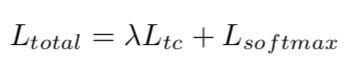Loss function has two terms. L_softmax optimizing a regular supervised classification problem. L_tc is a novel triple-center loss for enforcing a better embedding.

While most deep learning based approaches focus on leveraging the strong discriminative power of deep learning models for the classification of 3D data, only a few novel deep learning based approaches specifically designed for 3D object retrieval in large scale have been presented. In this post, I will review the new loss term (L_tc) which is the main paper contribution. It is not limited to multi-view 3D object retrieval application. Thus, the loss term and the following review are applicable to any retrieval problem.

For quick review, triple loss is the de facto standard loss for retrieval. Given a triple of anchor (A), positive sample (P) and negative sample (N), the triple loss promotes an embedding space where the distances between A,P is smaller than that of A,N by at least a margin m as shown in the following image.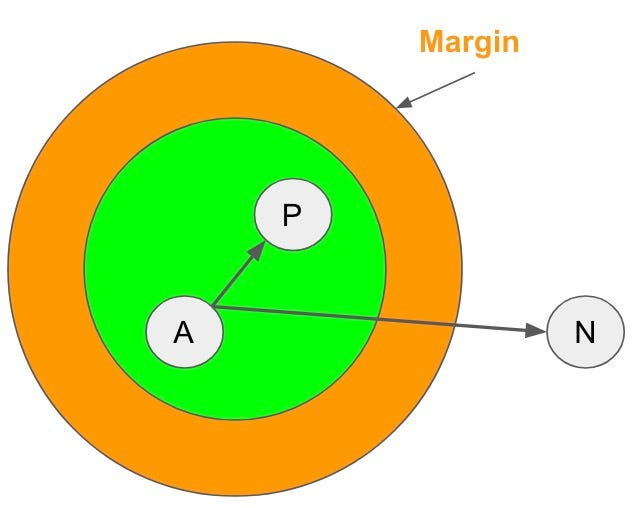The triple loss promotes an embedding space where the distances between A,P is smaller than that of A,N by at least a margin m

However, the number of triplets grows cubically when the training dataset gets larger, which usually results in a long impractical training period. Moreover, the performance of triplet loss highly relies on the mining of hard triplets, which is also time consuming. Meanwhile, how to define “good” hard triplets is still an open problem. All factors above make triplet loss hard to train. More details about triple loss can be found here.

A simple alternative for triple loss is center loss which pulls features/objects of the same class close to their corresponding center. While much easier to implement, the center loss has a degenerate solution. It is possible to embed all objects to a single point and this loss becomes zero.

In this paper, a two-fold approach is leveraged to avoid this degenerate solution. First, the retrieval center loss is trained along with the supervised classification softmax term. To classify objects, the softmax term must project different objects to different embedding. It serves as a good guider for seeking better class centers. While the degenerate solution is dodged, the current formulation, softmax + center loss, doesn’t push different centers from each other.

As shown in figure 2.b, center loss with softmax pull objects to their corresponding centers which is a desirable feature. Yet, It doesn’t maximize the margin between different classes centers. To tackle this problem, the paper proposes a new retrieval loss function called triplet-center loss (TCL). Given a pair of objects (P,N) and P’s corresponding center C, TCL promotes an embedding space where the distances between C,P is smaller than that of C,N by at least a margin m.

Thus, while Triplet loss formula is

Triplet Loss (A,P,N) ⇒ max(0, dist(A,P)-dist(A,N)+ margin)

The triplet-center loss (TCL) formula is

Triplet-center loss(P,N) ⇒ max(0, dist(C,P)-dist(C,N)+ margin) where C is P’s corresponding center.

As shown in figure 2.c, this novel triplet center loss formula ensures both that objects from the same class are closer to their center and different classes are distant from each other. The new TCL formulation is evaluated on benchmark datasets of 3D object retrieval, such as ModelNet40 and ShapeNet Core55.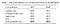softmax + triple center loss boost classification performance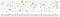T-SNE visualization for the embedding space using different loss functions. Softmax + triplet-center loss function (e) creates compact clusters that are distance from one another.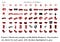Quantitative retrieval examples